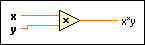# Multiply Function

## LabVIEW 2018 Help

Edition Date: March 2018
Part Number: 371361R-01
View Product InfoLabVIEW 2016 HelpLabVIEW 2017 HelpLabVIEW 2018 HelpLabVIEW 2019 HelpLabVIEW 2020 Help

Owning Palette: Numeric Functions

Requires: Base Development System

Returns the product of the inputs.

If you wire two waveform values or two dynamic data type values to this function, error in and error out terminals appear on the function. The connector pane displays the default data types for this polymorphic function.x can be a scalar number, array or cluster of numbers, array of clusters of numbers, and so on.y can be a scalar number, array or cluster of numbers, array of clusters of numbers, and so on.x*y is the product of x multiplied by y.Note  You can manually configure this function to output data of a type you want. To specify the output data type, right-click the function and select Properties to display the Object Properties dialog box. On the Output Configuration page, click the Representation icon and select the data type you want. A blue coercion dot appears on the output terminal of the function to indicate that you have configured the output data type.

## Multiply Details

You cannot use this function to multiply a matrix and a vector. Use the A x Vector VI instead.

When multiplying two matrices, if the number of rows in the second matrix does not match the number of columns in the first matrix, this function returns an empty matrix. Refer to the A x B VI for more information about matrix multiplication.

When you wire matrix data as an input to this function, a VI that includes subVIs that work with the matrix data type replaces the function. The resulting VI has the same icon but contains a matrix-specific algorithm. The node remains a VI if you disconnect the matrix from the input(s). Wire other data types as inputs to restore the original function. If you wire a data type to a function and that data type causes a basic math operation to fail, the function returns an empty matrix or NaN.

### Fixed-Point Details

If you wire fixed-point values to Numeric functions such as Add, Subtract, Multiply, and Square, the functions usually return values that do not lose any bits of word length. However, if the operation creates a value that exceeds the maximum word length that LabVIEW accepts, overflow or rounding conditions can occur. LabVIEW accepts a maximum word length of 64 bits. Use the Numeric Node Properties dialog box to configure how LabVIEW handles overflow or rounding of fixed-point data.

## Example

Refer to the Numeric Functions VI in the labview\examples\Numerics directory for an example of using the Multiply function.